Reasoning MCQ - 14

# Reasoning MCQ - 14 - Bank Exams

Test Description

## 30 Questions MCQ Test Reasoning Aptitude for Competitive Examinations - Reasoning MCQ - 14

Reasoning MCQ - 14 for Bank Exams 2023 is part of Reasoning Aptitude for Competitive Examinations preparation. The Reasoning MCQ - 14 questions and answers have been prepared according to the Bank Exams exam syllabus.The Reasoning MCQ - 14 MCQs are made for Bank Exams 2023 Exam. Find important definitions, questions, notes, meanings, examples, exercises, MCQs and online tests for Reasoning MCQ - 14 below.
Solutions of Reasoning MCQ - 14 questions in English are available as part of our Reasoning Aptitude for Competitive Examinations for Bank Exams & Reasoning MCQ - 14 solutions in Hindi for Reasoning Aptitude for Competitive Examinations course. Download more important topics, notes, lectures and mock test series for Bank Exams Exam by signing up for free. Attempt Reasoning MCQ - 14 | 30 questions in 30 minutes | Mock test for Bank Exams preparation | Free important questions MCQ to study Reasoning Aptitude for Competitive Examinations for Bank Exams Exam | Download free PDF with solutions
 1 Crore+ students have signed up on EduRev. Have you?
Reasoning MCQ - 14 - Question 1

### Directions : Study the following information carefully and answer the questions given below: Amartya Sen, Liu Xiaobo, Gerhard Domagk, Albert Fert, Andre Geim and Hideki Yukaw are six Nobel laureates, who belong to six different countries viz–India, China, Germany, France, Russia and Japan but not necessarily in the same order, each one has won the prize in different fields, viz Physics, Chemistry, Economics, Medicine, Peace and Literature but not necessarily in the same order. They are sitting around a circular table facing the centre. The persons who have won the prize for Medicine, Economics and Peace are neither from Japan nor from India. The persons who are from France and China have won the prizes neither for Medicine nor for Literature. Amartya Sen is neither from Japan nor sits on the immediate left of the person who won the prize for Chemistry. The only person who is between Andre Geim and Hideki Yukaw has won the prize for Physics. The person who is on the left side of the person from Japan has not won the prize for Literature. Albert Fert has won the prize for Chemistry and is from China. He is facing th  e person who has won the prize for Physics. One who has won the prize for Medicine is sitting opposite the person from Russia, while the person from China is on the left of the person who has won the prize for peace. One who has won the prize for Literature is on the immediate right of the person from Japan but on the left of the person who has won the prize for Economics. Gerhard Domagk has not won the prize for Economics and Hideki Yukaw has not won the prize for Medicine. Q. Who is Second To the left of Gerhard Domagk?

Detailed Solution for Reasoning MCQ - 14 - Question 1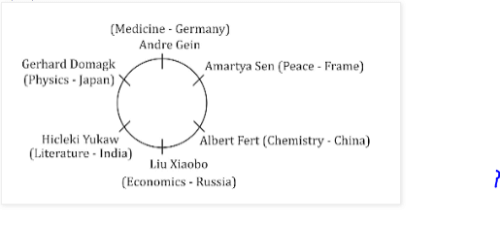Reasoning MCQ - 14 - Question 2

### Directions : Study the following information carefully and answer the questions given below: Amartya Sen, Liu Xiaobo, Gerhard Domagk, Albert Fert, Andre Geim and Hideki Yukaw are six Nobel laureates, who belong to six different countries viz–India, China, Germany, France, Russia and Japan but not necessarily in the same order, each one has won the prize in different fields, viz Physics, Chemistry, Economics, Medicine, Peace and Literature but not necessarily in the same order. They are sitting around a circular table facing the centre. The persons who have won the prize for Medicine, Economics and Peace are neither from Japan nor from India. The persons who are from France and China have won the prizes neither for Medicine nor for Literature. Amartya Sen is neither from Japan nor sits on the immediate left of the person who won the prize for Chemistry. The only person who is between Andre Geim and Hideki Yukaw has won the prize for Physics. The person who is on the left side of the person from Japan has not won the prize for Literature. Albert Fert has won the prize for Chemistry and is from China. He is facing th  e person who has won the prize for Physics. One who has won the prize for Medicine is sitting opposite the person from Russia, while the person from China is on the left of the person who has won the prize for peace. One who has won the prize for Literature is on the immediate right of the person from Japan but on the left of the person who has won the prize for Economics. Gerhard Domagk has not won the prize for Economics and Hideki Yukaw has not won the prize for Medicine. Q. Who likes Economics?

Detailed Solution for Reasoning MCQ - 14 - Question 2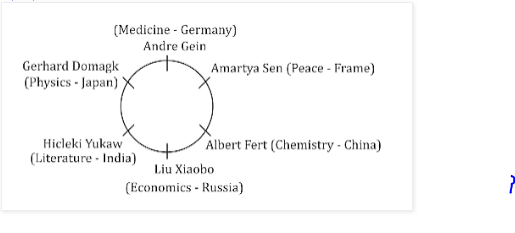Reasoning MCQ - 14 - Question 3

### Directions : Study the following information carefully and answer the questions given below: Amartya Sen, Liu Xiaobo, Gerhard Domagk, Albert Fert, Andre Geim and Hideki Yukaw are six Nobel laureates, who belong to six different countries viz–India, China, Germany, France, Russia and Japan but not necessarily in the same order, each one has won the prize in different fields, viz Physics, Chemistry, Economics, Medicine, Peace and Literature but not necessarily in the same order. They are sitting around a circular table facing the centre. The persons who have won the prize for Medicine, Economics and Peace are neither from Japan nor from India. The persons who are from France and China have won the prizes neither for Medicine nor for Literature. Amartya Sen is neither from Japan nor sits on the immediate left of the person who won the prize for Chemistry. The only person who is between Andre Geim and Hideki Yukaw has won the prize for Physics. The person who is on the left side of the person from Japan has not won the prize for Literature. Albert Fert has won the prize for Chemistry and is from China. He is facing th  e person who has won the prize for Physics. One who has won the prize for Medicine is sitting opposite the person from Russia, while the person from China is on the left of the person who has won the prize for peace. One who has won the prize for Literature is on the immediate right of the person from Japan but on the left of the person who has won the prize for Economics. Gerhard Domagk has not won the prize for Economics and Hideki Yukaw has not won the prize for Medicine. Q. Who is the third to the left of one who likes Medicine?

Detailed Solution for Reasoning MCQ - 14 - Question 3Reasoning MCQ - 14 - Question 4

Directions : Study the following information carefully and answer the questions given below:Amartya Sen, Liu Xiaobo, Gerhard Domagk, Albert Fert, Andre Geim and Hideki Yukaw are six Nobel laureates, who belong to six different countries viz–India, China, Germany, France, Russia and Japan but not necessarily in the same order, each one has won the prize in different fields, viz Physics, Chemistry, Economics, Medicine, Peace and Literature but not necessarily in the same order. They are sitting around a circular table facing the centre. The persons who have won the prize for Medicine, Economics and Peace are neither from Japan nor from India. The persons who are from France and China have won the prizes neither for Medicine nor for Literature. Amartya Sen is neither from Japan nor sits on the immediate left of the person who won the prize for Chemistry. The only person who is between Andre Geim and Hideki Yukaw has won the prize for Physics. The person who is on the left side of the person from Japan has not won the prize for Literature. Albert Fert has won the prize for Chemistry and is from China. He is facing th  e person who has won the prize for Physics. One who has won the prize for Medicine is sitting opposite the person from Russia, while the person from China is on the left of the person who has won the prize for peace. One who has won the prize for Literature is on the immediate right of the person from Japan but on the left of the person who has won the prize for Economics. Gerhard Domagk has not won the prize for Economics and Hideki Yukaw has not won the prize for Medicine.

Q.

Who among the following wins prize for Physics?

Detailed Solution for Reasoning MCQ - 14 - Question 4Reasoning MCQ - 14 - Question 5

Directions : Study the following information carefully and answer the questions given below:

Amartya Sen, Liu Xiaobo, Gerhard Domagk, Albert Fert, Andre Geim and Hideki Yukaw are six Nobel laureates, who belong to six different countries viz–India, China, Germany, France, Russia and Japan but not necessarily in the same order, each one has won the prize in different fields, viz Physics, Chemistry, Economics, Medicine, Peace and Literature but not necessarily in the same order. They are sitting around a circular table facing the centre. The persons who have won the prize for Medicine, Economics and Peace are neither from Japan nor from India. The persons who are from France and China have won the prizes neither for Medicine nor for Literature. Amartya Sen is neither from Japan nor sits on the immediate left of the person who won the prize for Chemistry. The only person who is between Andre Geim and Hideki Yukaw has won the prize for Physics. The person who is on the left side of the person from Japan has not won the prize for Literature. Albert Fert has won the prize for Chemistry and is from China. He is facing th  e person who has won the prize for Physics. One who has won the prize for Medicine is sitting opposite the person from Russia, while the person from China is on the left of the person who has won the prize for peace. One who has won the prize for Literature is on the immediate right of the person from Japan but on the left of the person who has won the prize for Economics. Gerhard Domagk has not won the prize for Economics and Hideki Yukaw has not won the prize for Medicine.

Q.

Which of the following combinations is true?

Detailed Solution for Reasoning MCQ - 14 - Question 5Reasoning MCQ - 14 - Question 6

Directions : Study the following information carefully and answer the questions given below:

Amartya Sen, Liu Xiaobo, Gerhard Domagk, Albert Fert, Andre Geim and Hideki Yukaw are six Nobel laureates, who belong to six different countries viz–India, China, Germany, France, Russia and Japan but not necessarily in the same order, each one has won the prize in different fields, viz Physics, Chemistry, Economics, Medicine, Peace and Literature but not necessarily in the same order. They are sitting around a circular table facing the centre. The persons who have won the prize for Medicine, Economics and Peace are neither from Japan nor from India. The persons who are from France and China have won the prizes neither for Medicine nor for Literature. Amartya Sen is neither from Japan nor sits on the immediate left of the person who won the prize for Chemistry. The only person who is between Andre Geim and Hideki Yukaw has won the prize for Physics. The person who is on the left side of the person from Japan has not won the prize for Literature. Albert Fert has won the prize for Chemistry and is from China. He is facing th  e person who has won the prize for Physics. One who has won the prize for Medicine is sitting opposite the person from Russia, while the person from China is on the left of the person who has won the prize for peace. One who has won the prize for Literature is on the immediate right of the person from Japan but on the left of the person who has won the prize for Economics. Gerhard Domagk has not won the prize for Economics and Hideki Yukaw has not won the prize for Medicine.

Q.

Hideki Yukaw is from which of the following country?

Detailed Solution for Reasoning MCQ - 14 - Question 6Reasoning MCQ - 14 - Question 7

Directions : Directions: In the following questions, two Conclusion is given and five statements are given as a), b), c), d) and e. From this you have to take the statements to be true even if they seem to be at variance with commonly known facts and then decide which of the given statement logically follows.

Q.

Conclusions:

All Donald being Micky is a possibility.

All Tom being Jerry is a possibility.

Detailed Solution for Reasoning MCQ - 14 - Question 7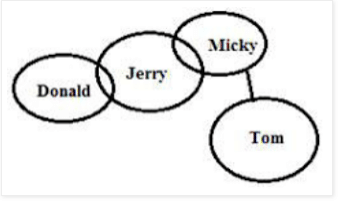Reasoning MCQ - 14 - Question 8

Directions : Directions: In the following questions, two Conclusion is given and five statements are given as a), b), c), d) and e. From this you have to take the statements to be true even if they seem to be at variance with commonly known facts and then decide which of the given statement logically follows.

Q.

Conclusions:

Some Table is plastic.

Some plastic are bench

Detailed Solution for Reasoning MCQ - 14 - Question 8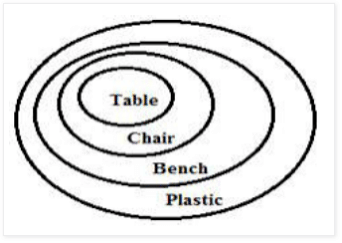Reasoning MCQ - 14 - Question 9

Directions : Directions: In the following questions, two Conclusion is given and five statements are given as a), b), c), d) and e. From this you have to take the statements to be true even if they seem to be at variance with commonly known facts and then decide which of the given statement logically follows.

Q.

Conclusions:

Some dates are day.

Some years are day.

Detailed Solution for Reasoning MCQ - 14 - Question 9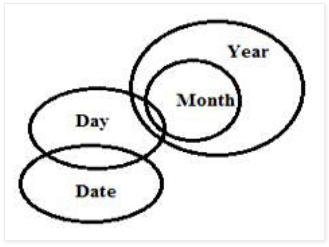Reasoning MCQ - 14 - Question 10

Directions : Directions: In the following questions, two Conclusion is given and five statements are given as a), b), c), d) and e. From this you have to take the statements to be true even if they seem to be at variance with commonly known facts and then decide which of the given statement logically follows.

Q.

Conclusions:

Some teachers are not student.

Some lessons are classroom.

Detailed Solution for Reasoning MCQ - 14 - Question 10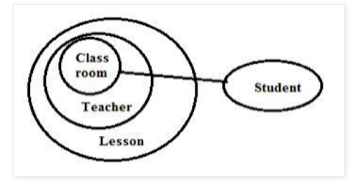Reasoning MCQ - 14 - Question 11

Directions : Directions: In the following questions, two Conclusion is given and five statements are given as a), b), c), d) and e. From this you have to take the statements to be true even if they seem to be at variance with commonly known facts and then decide which of the given statement logically follows.

Q.

Conclusions:

All windows being cot is a possibility.

Some doors are not pillow.

Detailed Solution for Reasoning MCQ - 14 - Question 11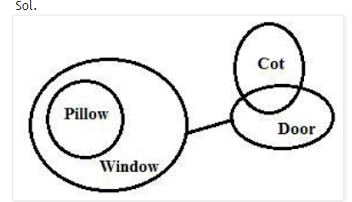Reasoning MCQ - 14 - Question 12

Directions : Consider the following statements and the questions that follow. We will analyse this problem in detail after that. In a certain code language

"login Mentors and check" is coded as "\$4e %4g #2n @6t"

"reads article study there" is coded as "@6i #4u \$4e %4a"

"comment about yours doubt" is coded as "\$4u @4o %6m #4u"

"get clear exams enjoy" is coded as "@4e #4a \$4j %2e"

Q.

What is the code for exams?

Reasoning MCQ - 14 - Question 13

Directions : Consider the following statements and the questions that follow. We will analyse this problem in detail after that. In a certain code language

"login Mentors and check" is coded as "\$4e %4g #2n @6t"

"reads article study there" is coded as "@6i #4u \$4e %4a"

"comment about yours doubt" is coded as "\$4u @4o %6m #4u"

"get clear exams enjoy" is coded as "@4e #4a \$4j %2e"

Q.

What is the code for study?

Reasoning MCQ - 14 - Question 14

Directions : Consider the following statements and the questions that follow. We will analyse this problem in detail after that. In a certain code language

"login Mentors and check" is coded as "\$4e %4g #2n @6t"

"reads article study there" is coded as "@6i #4u \$4e %4a"

"comment about yours doubt" is coded as "\$4u @4o %6m #4u"

"get clear exams enjoy" is coded as "@4e #4a \$4j %2e"

Q.

What is the code for about?

Reasoning MCQ - 14 - Question 15

Directions : Consider the following statements and the questions that follow. We will analyse this problem in detail after that. In a certain code language

"login Mentors and check" is coded as "\$4e %4g #2n @6t"

"reads article study there" is coded as "@6i #4u \$4e %4a"

"comment about yours doubt" is coded as "\$4u @4o %6m #4u"

"get clear exams enjoy" is coded as "@4e #4a \$4j %2e"

Q.

If we code "study Mentors clear exams" as per the above coding method, what will be the code?

Reasoning MCQ - 14 - Question 16

Directions : In the following questions, the symbols \$, @, £, • and # are used with the following meanings as illustrated below:

‘A \$ B' means A is neither greater nor smaller than B

‘A @ B' means A is neither greater than nor equal to B

`A £ B' means A is neither smaller than nor equal to B

‘A • B' means A is not smaller than B

‘A # B' means A is not greater than B

In each of the following questions, assuming the given statements to be true, find out which of the two conclusions I and II given below them is/are definitely true. Give answer

Q.

Statements: W • P,  P £ G,  G @ I,  I # N Conclusions: I. I £ P  II. N # W

Reasoning MCQ - 14 - Question 17

Directions : In the following questions, the symbols \$, @, £, • and # are used with the following meanings as illustrated below:

‘A \$ B' means A is neither greater nor smaller than B

‘A @ B' means A is neither greater than nor equal to B

`A £ B' means A is neither smaller than nor equal to B

‘A • B' means A is not smaller than B

‘A # B' means A is not greater than B

In each of the following questions, assuming the given statements to be true, find out which of the two conclusions I and II given below them is/are definitely true. Give answer

Q.

Statements: U @ D,  D \$ E,  E £ Y,  Y • W

Conclusions: I. W @ E  II. D £ W

Reasoning MCQ - 14 - Question 18

Directions : In the following questions, the symbols \$, @, £, • and # are used with the following meanings as illustrated below:

‘A \$ B' means A is neither greater nor smaller than B

‘A @ B' means A is neither greater than nor equal to B

`A £ B' means A is neither smaller than nor equal to B

‘A • B' means A is not smaller than B

‘A # B' means A is not greater than B

In each of the following questions, assuming the given statements to be true, find out which of the two conclusions I and II given below them is/are definitely true. Give answer

Q.

Statements: Z £ N,  N # K,  K \$ M,  M @ R

Conclusions: I. M \$ N  II. M £  N

Reasoning MCQ - 14 - Question 19

Directions : In the following questions, the symbols \$, @, £, • and # are used with the following meanings as illustrated below:

‘A \$ B' means A is neither greater nor smaller than B

‘A @ B' means A is neither greater than nor equal to B

`A £ B' means A is neither smaller than nor equal to B

‘A • B' means A is not smaller than B

‘A # B' means A is not greater than B

In each of the following questions, assuming the given statements to be true, find out which of the two conclusions I and II given below them is/are definitely true. Give answer

Q.

Statements: V • D,  D £ T,  K \$ T,  K # F

Conclusions: I. D £  K  II. T • F

Reasoning MCQ - 14 - Question 20

Directions : In the following questions, the symbols \$, @, £, • and # are used with the following meanings as illustrated below:

‘A \$ B' means A is neither greater nor smaller than B

‘A @ B' means A is neither greater than nor equal to B

`A £ B' means A is neither smaller than nor equal to B

‘A • B' means A is not smaller than B

‘A # B' means A is not greater than B

In each of the following questions, assuming the given statements to be true, find out which of the two conclusions I and II given below them is/are definitely true. Give answer

Q.

Statements: S \$ Q,  Q @ B,  B • K,  K # W

Conclusions: I. K # S  II. S @ W

Reasoning MCQ - 14 - Question 21

Directions : In the following questions the symbols @, +, ©, \$, ∆  and ? are used with the following meaning:

P ∆ Q means P is not equal to Q.

P @ Q means P is greater than Q.

P + Q means P is smaller than Q.

P © Q means P is either greater than or equal to Q.

P \$ Q means P is either smaller than or equal to Q.

P ? Q means P is equal to Q.

Now in each of the following questions assuming the given statements to be true, ﬁnd which of the two conclusions I and ll given below them is/are deﬁnitely true. Give answer.

Reasoning MCQ - 14 - Question 22

Directions : In the following questions the symbols @, +, ©, \$, ∆  and ? are used with the following meaning:

P ∆ Q means P is not equal to Q.

P @ Q means P is greater than Q.

P + Q means P is smaller than Q.

P © Q means P is either greater than or equal to Q.

P \$ Q means P is either smaller than or equal to Q.

P ? Q means P is equal to Q.

Now in each of the following questions assuming the given statements to be true, ﬁnd which of the two conclusions I and ll given below them is/are deﬁnitely true. Give answer

Q.

Statements:K © M,     M ∆ R,    R ? T

Conclusions: I. K © T  II. M ? T

Reasoning MCQ - 14 - Question 23

Directions : In the following questions the symbols @, +, ©, \$, ∆  and ? are used with the following meaning:

P ∆ Q means P is not equal to Q.

P @ Q means P is greater than Q.

P + Q means P is smaller than Q.

P © Q means P is either greater than or equal to Q.

P \$ Q means P is either smaller than or equal to Q.

P ? Q means P is equal to Q.

Now in each of the following questions assuming the given statements to be true, ﬁnd which of the two conclusions I and ll given below them is/are deﬁnitely true. Give answer

Q.

Statements: B+D,      D@N,    N\$H

Reasoning MCQ - 14 - Question 24

Directions : In the following questions the symbols @, +, ©, \$, ∆  and ? are used with the following meaning:

P ∆ Q means P is not equal to Q.

P @ Q means P is greater than Q.

P + Q means P is smaller than Q.

P © Q means P is either greater than or equal to Q.

P \$ Q means P is either smaller than or equal to Q.

P ? Q means P is equal to Q.

Now in each of the following questions assuming the given statements to be true, ﬁnd which of the two conclusions I and ll given below them is/are deﬁnitely true. Give answer

Q.

Conclusions: I. M@N  II.M?N

Reasoning MCQ - 14 - Question 25

Directions : In the following questions the symbols @, +, ©, \$, ∆  and ? are used with the following meaning:

P ∆ Q means P is not equal to Q.

P @ Q means P is greater than Q.

P + Q means P is smaller than Q.

P © Q means P is either greater than or equal to Q.

P \$ Q means P is either smaller than or equal to Q.

P ? Q means P is equal to Q.

Now in each of the following questions assuming the given statements to be true, ﬁnd which of the two conclusions I and ll given below them is/are deﬁnitely true. Give answer

Q.

Statements: T\$M,     M?Q,     Q+R

Conclusions: I. Q @ T  II.Q ? T

Reasoning MCQ - 14 - Question 26

Directions : In the following questions the symbols @, +, ©, \$, ∆  and ? are used with the following meaning:

P ∆ Q means P is not equal to Q.

P @ Q means P is greater than Q.

P + Q means P is smaller than Q.

P © Q means P is either greater than or equal to Q.

P \$ Q means P is either smaller than or equal to Q.

P ? Q means P is equal to Q.

Now in each of the following questions assuming the given statements to be true, ﬁnd which of the two conclusions I and ll given below them is/are deﬁnitely true. Give answer

Q.

Statements: D @ B,   B\$T,    T + M

Reasoning MCQ - 14 - Question 27

Directions:  In the following questions, the symbols @, \$, %, # and © are used with the following meanings illustrated—

(i) ‘X @ Y’ means ‘X is not smaller than Y’.

(ii) ‘X \$ Y’ means ‘X is not greater than Y’.

(iii) ‘X % Y’ means ‘X is neither smaller than nor equal to Y’.

(iv) ‘X # Y’ means ‘X is neither greater than nor equal to Y’.

(v) ‘X © Y’ means ‘X is neither smaller than nor greater than Y’.

In each of the following questions assuming the given statements to be true, find out which of the two conclusions I and II given below them is/are definitely true. Give answer

Q.

Statements:  P \$ T,  T @ L,   U % L

Conclusions: I. P @ L II. U©L

Reasoning MCQ - 14 - Question 28

Directions:  In the following questions, the symbols @, \$, %, # and © are used with the following meanings illustrated—

(i) ‘X @ Y’ means ‘X is not smaller than Y’.

(ii) ‘X \$ Y’ means ‘X is not greater than Y’.

(iii) ‘X % Y’ means ‘X is neither smaller than nor equal to Y’.

(iv) ‘X # Y’ means ‘X is neither greater than nor equal to Y’.

(v) ‘X © Y’ means ‘X is neither smaller than nor greater than Y’.

In each of the following questions assuming the given statements to be true, find out which of the two conclusions I and II given below them is/are definitely true. Give answer

Q.

Reasoning MCQ - 14 - Question 29

Directions:  In the following questions, the symbols @, \$, %, # and © are used with the following meanings illustrated—

(i) ‘X @ Y’ means ‘X is not smaller than Y’.

(ii) ‘X \$ Y’ means ‘X is not greater than Y’.

(iii) ‘X % Y’ means ‘X is neither smaller than nor equal to Y’.

(iv) ‘X # Y’ means ‘X is neither greater than nor equal to Y’.

(v) ‘X © Y’ means ‘X is neither smaller than nor greater than Y’.

In each of the following questions assuming the given statements to be true, find out which of the two conclusions I and II given below them is/are definitely true. Give answer.

Q.

Statements:  J # K,   K©L,   P\$L

Conclusions: I. P\$K II. J#L

Reasoning MCQ - 14 - Question 30

Directions:  In the following questions, the symbols @, \$, %, # and © are used with the following meanings illustrated—

(i) ‘X @ Y’ means ‘X is not smaller than Y’.

(ii) ‘X \$ Y’ means ‘X is not greater than Y’.

(iii) ‘X % Y’ means ‘X is neither smaller than nor equal to Y’.

(iv) ‘X # Y’ means ‘X is neither greater than nor equal to Y’.

(v) ‘X © Y’ means ‘X is neither smaller than nor greater than Y’.

In each of the following questions assuming the given statements to be true, find out which of the two conclusions I and II given below them is/are definitely true. Give answer

Q.

Statements: T % O,  T # M,   M@ V

Conclusions: I. M % T II. O % V

## Reasoning Aptitude for Competitive Examinations

11 videos|20 docs|171 tests
Information about Reasoning MCQ - 14 Page
In this test you can find the Exam questions for Reasoning MCQ - 14 solved & explained in the simplest way possible. Besides giving Questions and answers for Reasoning MCQ - 14, EduRev gives you an ample number of Online tests for practice

## Reasoning Aptitude for Competitive Examinations

11 videos|20 docs|171 tests